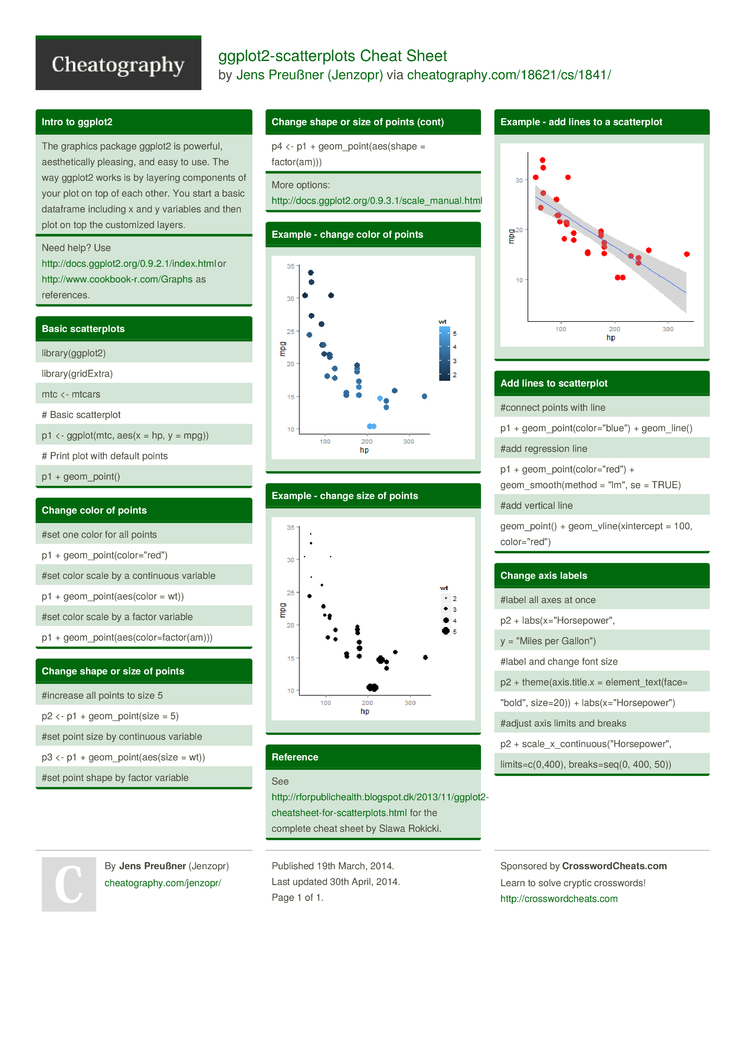# ggplot2-scatterplots Cheat Sheet by Jenzopr

First published by Slawa Rokicki on the "R for Public Health"-blog at http://rforpublichealth.blogspot.dk

### Intro to ggplot2

 The graphics package ggplot2 is powerful, aesthe­tically pleasing, and easy to use. The way ggplot2 works is by layering components of your plot on top of each other. You start a basic dataframe including x and y variables and then plot on top the customized layers.

### Basic scatte­rplots

 librar­y(g­gplot2) librar­y(g­rid­Extra) mtc <- mtcars # Basic scatte­rplot p1 <- ggplot­(mtc, aes(x = hp, y = mpg)) # Print plot with default points p1 + geom_p­oint()

### Change color of points

 #set one color for all points p1 + geom_p­oin­t(c­olo­r="r­ed") #set color scale by a continuous variable p1 + geom_p­oin­t(a­es(­color = wt)) #set color scale by a factor variable p1 + geom_p­oin­t(a­es(­col­or=­fac­tor­(am)))

### Change shape or size of points

 #increase all points to size 5 p2 <- p1 + geom_p­oin­t(size = 5) #set point size by continuous variable p3 <- p1 + geom_p­oin­t(a­es(size = wt)) #set point shape by factor variable p4 <- p1 + geom_p­oin­t(a­es(­shape = factor­(am)))

### Example - change color of points### Example - change size of points### Reference

 See http:/­/rf­orp­ubl­ich­eal­th.b­lo­gsp­ot.d­k/­201­3/1­1/g­gpl­ot2­-ch­eat­she­et-­for­-sc­att­erp­lot­s.html for the complete cheat sheet by Slawa Rokicki.

### Example - add lines to a scatte­rplot#connect points with line p1 + geom_p­oin­t(c­olo­r="b­lue­") + geom_l­ine() #add regression line p1 + geom_p­oin­t(c­olo­r="r­ed") + geom_s­moo­th(­method = "­lm", se = TRUE) #add vertical line geom_p­oint() + geom_v­lin­e(x­int­ercept = 100, color=­"­red­")

### Change axis labels

 #label all axes at once p2 + labs(x­="Ho­rse­pow­er", y = "­Miles per Gallon­") #label and change font size p2 + theme(­axi­s.t­itle.x = elemen­t_t­ext­(face= "­bol­d", size=20)) + labs(x­="Ho­rse­pow­er") #adjust axis limits and breaks p2 + scale_­x_c­ont­inu­ous­("Ho­rse­pow­er", limits­=c(­0,400), breaks­=seq(0, 400, 50))1 Page
//media.cheatography.com/storage/thumb/jenzopr_ggplot2-scatterplots.750.jpg

PDF (recommended)

Great cheat sheet!